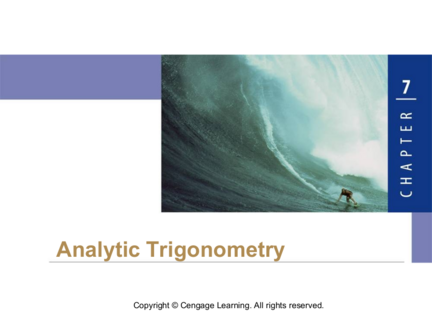# Basic Trigonometric EquationsContributed by:OBJECTIVES:
1. Basic Trigonometric Equations
2. Solving Trigonometric Equations by Factoring
1. Analytic Trigonometry
2. Basic Trigonometric
Equations
3. ► Basic Trigonometric Equations
► Solving Trigonometric Equations by
Factoring
3
4. Basic Trigonometric Equations
4
5. Basic Trigonometric Equations
An equation that contains trigonometric functions is called a
trigonometric equation. For example, the following are
trigonometric equations:
sin2  + cos2  = 1 2 sin – 1 = 0 tan 2 – 1 = 0
The first equation is an identity—that is, it is true for every
value of the variable . The other two equations are true
only for certain values of .
To solve a trigonometric equation, we find all the values of
the variable that make the equation true.
5
6. Basic Trigonometric Equations
Solving any trigonometric equation always reduces to
solving a basic trigonometric equation—an equation of
the form Trig( ) = c, where Trig is a trigonometric function
and c is a constant. For example:
2 sin  – 1 = 0
In the next example we solve such basic equations.
6
7. Example – Solving Trigonometric Equations
Find all solutions of the equation.
(a)2 sin  – 1 = 0
(a) We start by isolating sin  :
2 sin  – 1 = 0 Given equation
2 sin  = 1
Divide by 2
sin  =
Now use the table at the bottom of the identity formula sheet and look to see
Where sin equals 1/2
7
8. Example – Solution cont’d
This time we must consider that since sine is positive in the
first and second quadrants, the solutions are
= + 2k = + 2k
where k is any integer.
8
9. Example – Solution cont’d
(b) tan2  – 3 = 0
We start by isolating tan  :
tan2  – 3 = 0 Given equation
tan2  = 3 Add 3
tan  Take the square root
9
10. Example – Solution cont’d
Because tangent is positive in both QI & QIII and negative
in QII & QIV, first find one value for the positive tan and
then one value for the negative tan and finally to get all
solutions, we add integer multiples of  or 180 º to these
 = + k =2 + k
OR in Degrees:
 = 60º + 180k  = 120º + 180k
where k is any integer.
10
11. Solving Trigonometric Equations
by Factoring
11
12. Solving Trigonometric Equations by Factoring
12
13. Example – A Trigonometric Equation of Quadratic Type
Now solve the equation 2 cos2  – 7 cos  + 3 = 0.
We factor the left-hand side of the equation.
2 cos2  – 7 cos  + 3 = 0 Given equation
(2 cos  – 1)(cos  – 3) = 0 Factor
2 cos  – 1 = 0 or cos  – 3 = 0 Set each factor equal to 0
cos  = or cos  = 3 Solve for cos 
13
14. Example – Solution cont’d
Because cosine has period 2, we first find the solutions in
the interval [0, 2). For the first equation the solutions are
 =  /3 and  = 5 /3 (see Figure 7 and your formula
Figure 7
14
15. Example – Solution cont’d
The second equation has no solution because cos  is
never greater than 1.
Thus the solutions are
= + 2k = + 2k
where k is any integer.
15
16. Example – Solving a Trigonometric Equation by Factoring
Given equation
Factor
Set each factor equal to 0
Solve for sin 
16
17. Example – Solution cont’d
Because sine and cosine have period 2 or 360º we first
find the solutions of these equations in an interval of length
For the first equation cos  = 0 ,
the solutions in the interval [0, 360º) are  = 90º and
 = 270º. To solve the second equation, we take sin–1 of
each side:
sin  = –0.80
Second equation
 = sin–1(–0.80) Take sin–1 of each side with your
calculator let’s use degree mode
17
18. Example – Solution cont’d
  - 53.13 º Calculator (in degree mode)
So the solutions in an interval of length 360ºare  = - 53.13 º
and then since sine is also negative in the third quadrant,
 = 180 + 53.13 or 233.13º
18
19. Example – Solution cont’d
We get all the solutions of the equation by adding integer
multiples of 360º to these solutions.
 = 90 + 360k,  = 270 +360k
  – 53.13 + 360k   233.13 + 360k
where k is any integer.
19